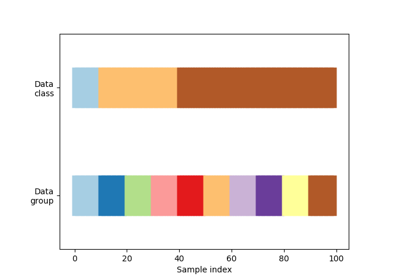# sklearn.model_selection.TimeSeriesSplit¶

class sklearn.model_selection.TimeSeriesSplit(n_splits=5, max_train_size=None)[source]

Time Series cross-validator

Provides train/test indices to split time series data samples that are observed at fixed time intervals, in train/test sets. In each split, test indices must be higher than before, and thus shuffling in cross validator is inappropriate.

This cross-validation object is a variation of KFold. In the kth split, it returns first k folds as train set and the (k+1)th fold as test set.

Note that unlike standard cross-validation methods, successive training sets are supersets of those that come before them.

Read more in the User Guide.

Parameters
n_splitsint, default=5

Number of splits. Must be at least 2.

Changed in version 0.22: n_splits default value changed from 3 to 5.

max_train_sizeint, optional

Maximum size for a single training set.

Notes

The training set has size i * n_samples // (n_splits + 1) + n_samples % (n_splits + 1) in the ith split, with a test set of size n_samples//(n_splits + 1), where n_samples is the number of samples.

Examples

>>> import numpy as np
>>> from sklearn.model_selection import TimeSeriesSplit
>>> X = np.array([[1, 2], [3, 4], [1, 2], [3, 4], [1, 2], [3, 4]])
>>> y = np.array([1, 2, 3, 4, 5, 6])
>>> tscv = TimeSeriesSplit()
>>> print(tscv)
TimeSeriesSplit(max_train_size=None, n_splits=5)
>>> for train_index, test_index in tscv.split(X):
...     print("TRAIN:", train_index, "TEST:", test_index)
...     X_train, X_test = X[train_index], X[test_index]
...     y_train, y_test = y[train_index], y[test_index]
TRAIN:  TEST: 
TRAIN: [0 1] TEST: 
TRAIN: [0 1 2] TEST: 
TRAIN: [0 1 2 3] TEST: 
TRAIN: [0 1 2 3 4] TEST: 


Methods

 get_n_splits(self[, X, y, groups]) Returns the number of splitting iterations in the cross-validator split(self, X[, y, groups]) Generate indices to split data into training and test set.
__init__(self, n_splits=5, max_train_size=None)[source]

Initialize self. See help(type(self)) for accurate signature.

get_n_splits(self, X=None, y=None, groups=None)[source]

Returns the number of splitting iterations in the cross-validator

Parameters
Xobject

Always ignored, exists for compatibility.

yobject

Always ignored, exists for compatibility.

groupsobject

Always ignored, exists for compatibility.

Returns
n_splitsint

Returns the number of splitting iterations in the cross-validator.

split(self, X, y=None, groups=None)[source]

Generate indices to split data into training and test set.

Parameters
Xarray-like, shape (n_samples, n_features)

Training data, where n_samples is the number of samples and n_features is the number of features.

yarray-like, shape (n_samples,)

Always ignored, exists for compatibility.

groupsarray-like, with shape (n_samples,)

Always ignored, exists for compatibility.

Yields
trainndarray

The training set indices for that split.

testndarray

The testing set indices for that split.

## Examples using sklearn.model_selection.TimeSeriesSplit¶